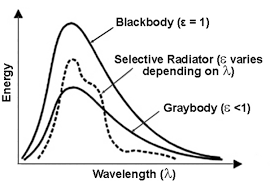## How to Calculate and Solve for Monochromatic Emissive Power | Radiation Heat TransferThe image above represents monochromatic emissive power.

To compute for monochromatic emissive power, five essential parameters are needed and these parameters are Planck’s Constant (h), Velocity of Light (c), Wavelength (λ), Boltzmann’s Constant (KB) and Temperature (T).

The formula for calculating monochromatic emissive power:

ebx = 2πhc²λ-5 / exp(ch/KBλT) – 1

Where:

ebx = Monochromatic Emissive Power | Planck’s Equation
h = Planck’s Constant
c = Velocity of Light
λ = Wavelength
KB = Boltzmann’s Constant
T = Temperature

Let’s solve an example;
Find the monochromatic emissive power when the planck’s constant is 6.626E-24, the velocity of light is 3E8, the wavelength is 22, the boltzmann’s constant is 1.380E-23 and the temperature is 10.

This implies that;

h = Planck’s Constant = 6.626E-24
c = Velocity of Light = 3E8
λ = Wavelength = 22
KB = Boltzmann’s Constant = 1.380E-23
T = Temperature = 10

ebx = 2πhc²λ-5 / exp(ch/KBλT) – 1
ebx = 2π(6.62607004e-34)(300000000)²(22)-5 / exp((300000000)(6.62607004e-34)/(1.38064852e-23)(22)(10)) – 1
ebx = 2π(6.62607004e-34)(90000000000000000)(1.94037e-7) / exp(1.987821012e-25/3.037426744e-21) – 1
ebx = 7.270512005456302e-23 / exp(0.00006544424539379114) – 1
ebx = 7.270512005456302e-23 / 1.0000654463869152 – 1
ebx = 7.270512005456302e-23 / 0.00006544638691519111
ebx = 1.11e-18

Therefore, the monochromatic emissive power is 1.11e-18.